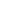# Design Consideration Of Tension Members

Tension members, as described in IS 800:2007, can sustain loads up to the ultimate load, at which stage they may fail by rupture at a critical section. However, if the gross area of the member yields over a major portion of its length before the rupture load is reached, the member may become non-functional due to excessive elongation. Plates and other rolled sections in tension may also fail by block shear of end bolted regions also. The design objective is basically to check these three failure modes under prescribed loads or combination of loads.

DESIGN CONSIDERATION OF TENSION MEMBERS

The factored design tension, T, in the members shall satisfy T<=Td as detailed in Cl. 6.1 of IS 800:2007 where Td = design strength of the member. The design strength of a member under axial tension,Td ,  is  the  lowest  of  the  design  strength  due to  yielding of  gross  section ,rupture  strength of critical section (Tdn ),and block shear strength (Tdp), given in clauses 6.2, 6.3 and 6.4 of IS 800:2007 respectively.

1. Design Strength due to Yielding of Gross Section

The design strength of members under axial tension  as governed by yielding of gross section, as per IS 800:2007,  is given by  Ag/ty/rmo where  is the yield stress of the material, Ag is the gross area of cross-section, and rmo is the partial safety factor for failure in tension by yielding as given in Table 5 of IS 800:2007. This table is reproduced here as Table 8.

Table 8 Partial Safety Factors for Materials

Sl No          Definition                      Partial safety factor

1        Resistance, governed by yielding, ??                   1.10

2        Resistance of member to buckling, ??                  1.10

3        Resistance, governed by ultimate stress, ??                  1.25

4        Resistance of connection                   Shop Fabrications         Field Fabrications

4(a)   Bolts-Friction Type, ??                    1.25   1.25

4(b)   Bolts-Bearing Type, ???                            1.25   1.25

4(c)    Rivets,        ???                      1.25   1.25

4(d)   Welds,        ??                         1.25   1.50

2. Design Strength due to Rupture Strength of Critical Section

The rupture strength of the critical section is also a governing criterion for the design of tension members. Cl. 6.3 of IS 800:2007 deals with the rupture strength of critical section. The critical section has to be carefully evaluated as the section which is having the least net cross sectional area out of the various possible failure planes. The evaluation of the strength of critical sections depends on the type of cross section. The calculation will be based on Cl. 6.3.1 (Plates), Cl. 6.3.2 (Threaded rods), Cl.

6.3.3 (Angles) and Cl. 6.3.4 (Other sections) of IS 800:2007. Our discussion will be limited to that of plates and angles in this section.

2.1. Design Strength due to Rupture Strength of Critical Section for plates

Cl. 6.3.1 of IS 800:2007 gives the design strength in tension of a plate, Tdn, as governed by rupture of net cross-sectional area, An, at the holes is Tdn= 0.9Anfu/rms where rms is the partial safety factor for failure at ultimate stress as given in Table 8 above or Table 5 of IS 800:2007, An is the ultimate stress of the material, andis the net effective area of the member given by,| b-ndn + Ew| t where b and t  are  the width and thickness of the plate, dh is the diameter of the bolt hole (2 mm in addition to the diameter of the hole, in case the directly punched holes), g is the

gauge length between the bolt holes, as shown in Fig. 5 of IS 800:2007. This figure is reproduced here as Figure 27. ‘p’ is the staggered-pitch length between line of bolt holes, as shown in Fig. 5 of IS

800:2007, n is the number of bolt holes in the critical section and i is the subscript for summation of all the inclined legs.

2.2. Design Strength due to Rupture Strength of Critical Section for plates

Cl. 6.3.3 of IS 800:2007 gives the rupture strength of angles connected through one leg. This strength will be affected by shear lag and is given by Tdn. This should be limited by a maximum value given by and by a minimum value of 0.7 where w is the outstand leg width, bs is the shear lag width, as shown in Fig. 6 of IS800:2007 (Reproduced here as Figure 28), Lcis the length of the end connection, that is the distance between the outermost bolts in the end joint measured along the load direction or length of the weld along the load direction, An is the net area of the total cross-section, Ago is the gross area of the outstanding leg, Anc is the net area of the connected leg and t is the thickness of the leg.

In the case of design of tension members, the IS800:2007 permits to use a simpler expression for preliminary sizing. The approximate rupture strength of net section to be taken as Tdn = aAnfu/rms where acan be taken as 0.6 for one or two bolts, 0.7 for three bolts and 0.8 for four or more bolts along the length in the end connection or equivalent weld length.

2.3. Design Strength due to Rupture Strength of Critical Section for Other Section

According to Cl. 6.3.4 of IS800:2007, the rupture strength,, of the double angles, channels, I-sections and other rolled steel sections, connected by one or more elements to an end gusset is also governed by shear lag effects. The design tensile strength of such sections as governed by tearing of net section may also be calculated using equation in 6.3.3, where is calculated based on the shear lag distance,  , taken from the farthest edge of the outstanding leg to the nearest bolt/weld line in the connected leg of the cross-section.